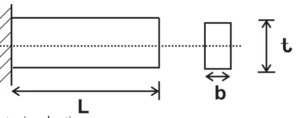Engineering Jobs   »   Mechanical Engineering Quiz

# UPRVUNL’21 ME: Daily Practices Quiz. 6-Sep-2021

Quiz: Mechanical Engineering
Exam: UPRVUNL
Topic: Miscellaneous

Each question carries 1 mark
Negative marking: 1/4 mark
Time: 8 Minutes

Q1. For a long and narrow cross-section (i.e., ratio of b/t, breadth b and thickness t above 10) bar subjected to torsion T, the value of maximum shear stress will be)
(a) T/bt²
(b) T/ (4bt²)
(c) 2T/bt²
(d) None of these

Q2. The thread used for transmission of power is

Q3. The number of instantaneous centres for the mechanism having 5 links is…………
(a) 6
(b) 4
(c) 12
(d) 10

Q4. For a slider crank mechanism, the velocity and acceleration of the piston at inner dead Centre will be:
Where ‘ω’ is the angular velocity of the crank and ‘r’ its radius.
(a) 0 and 0
(b) 0 and ω² r
(c) 0 and < ω² r
(d) 0 and > ω² r

Q5. Which of the following is not an extensive property?
(a) entropy
(b) enthalpy
(c) internal energy
(d) density

Q6. Properties of substances like pressure, temperature and density in thermodynamic coordinates are
(a) path functions
(b) point functions
(c) cyclic functions
(d) real functions

Solutions
S1. Ans.(d)
Sol.from torsion education
T/J=τ/r
τ=Tr/J
= (T×t/2)/((bt^3)/6)
▭(τ_max=τ=3T/(bt^2 ))

S2. Ans.(a)
Sol. for power transmission square threads are used as it has high efficiency. Applications of square threads are screw Jack, power transmission mechanism etc.
S3. Ans.(d)
Sol. We know,
No. of instantaneous center(N) = n(n-1)/2 ——— (1)

From eqn. (1)
N=5(5-1)/2
▭(N=10)

S4. Ans.(d)
Sol.

we know that,
In a slider-crank mechanism,

At, inner dead center θ = 0°
Velocity of slider (v) = rω(sinθ+sin2θ/2n)
= 0
At θ=0°
Acceleration of slider (a) = r=rω^2 (cosθ+cos2θ/n)
=rω^2 ( 1+1/n)>rω^2 orω^2 r
S5. Ans.(d)
Sol.
Properties represents the characteristics of the system. Properties which depend on mass is called extensive or extrinsic properties.
Example : Mass, volume entropy, enthalpy, internal energy.

S6. Ans.(b)
Sol.
Properties of substances like pressure, temperature and density in thermodynamic coordinates are point functions.

Sharing is caring!